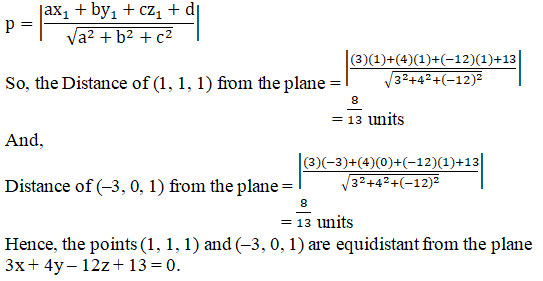# RD Sharma Solutions Class 12 The Plane Exercise 29.9

RD Sharma Solutions for Class 12 Maths Chapter 29 The Plane Exercise 29.9, is given here. Students who aim to secure top scores in their examinations can refer to RD Sharma Solutions, which is the best reference material prepared by our experts, keeping in mind the latest syllabus. Practising these RD Sharma Class 12 Solutions will ensure that they can easily excel in their final exams. The pdf consisting of this chapter solutions which are available for free can be downloaded from the links given below.

## Download PDF of RD Sharma Solutions For Class 12 Maths Chapter 29 Exercise 9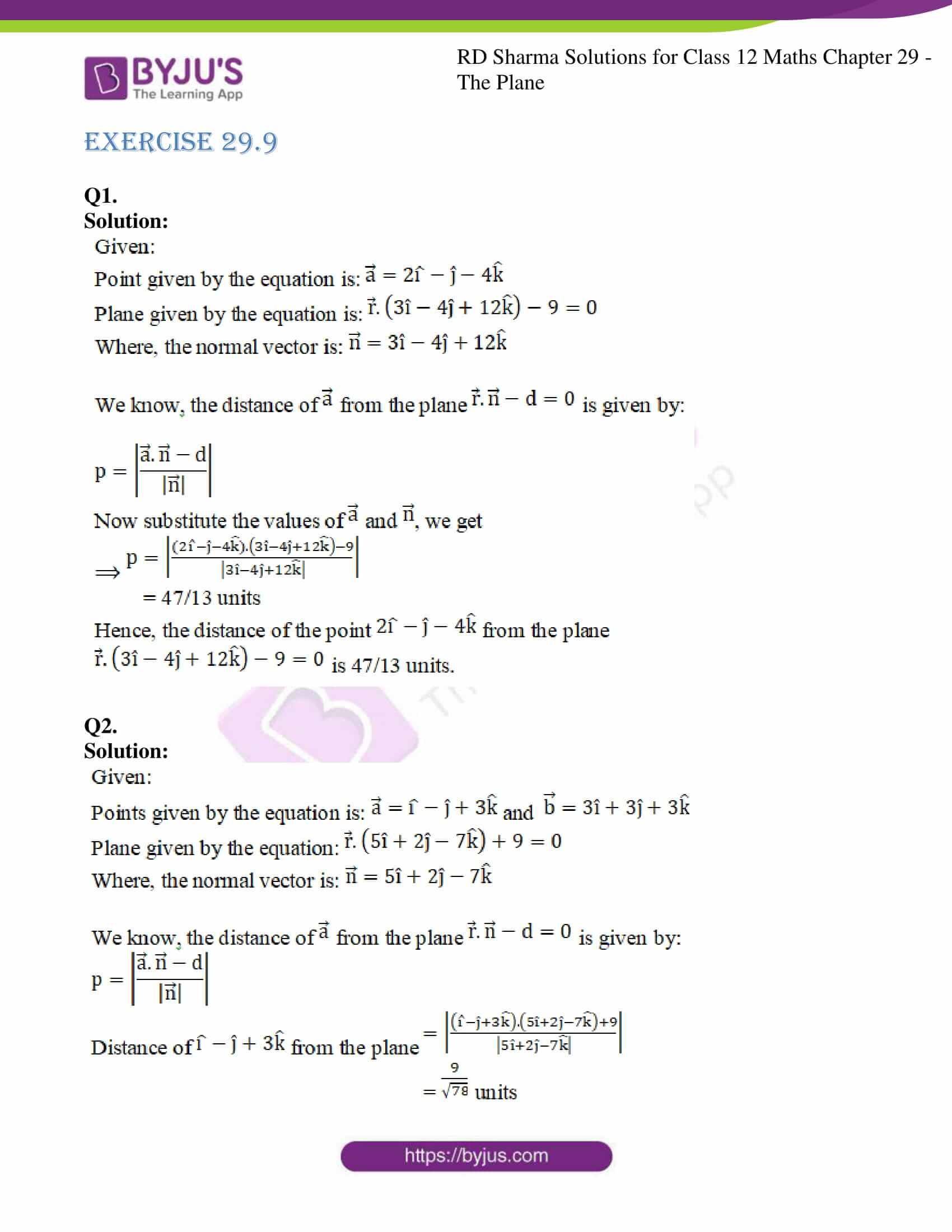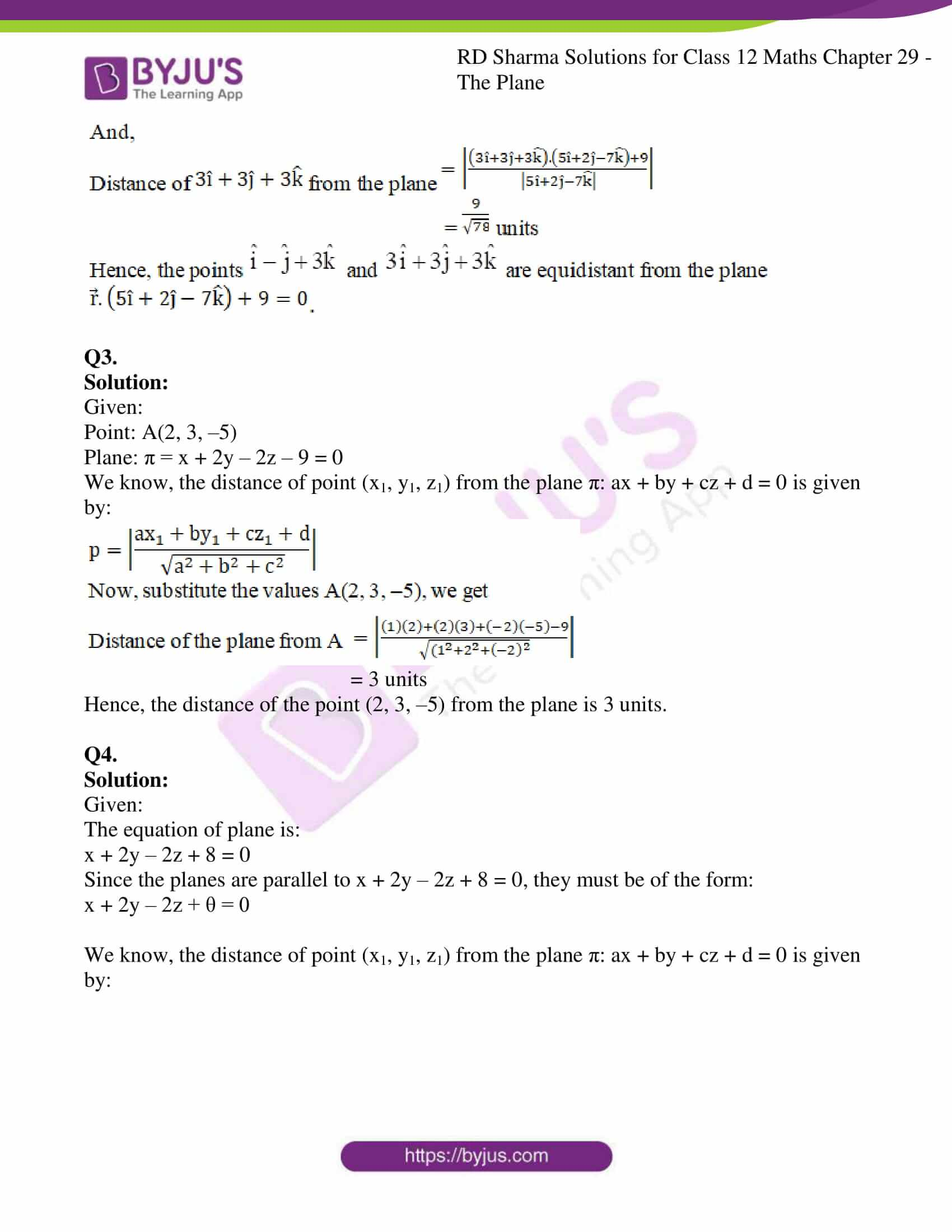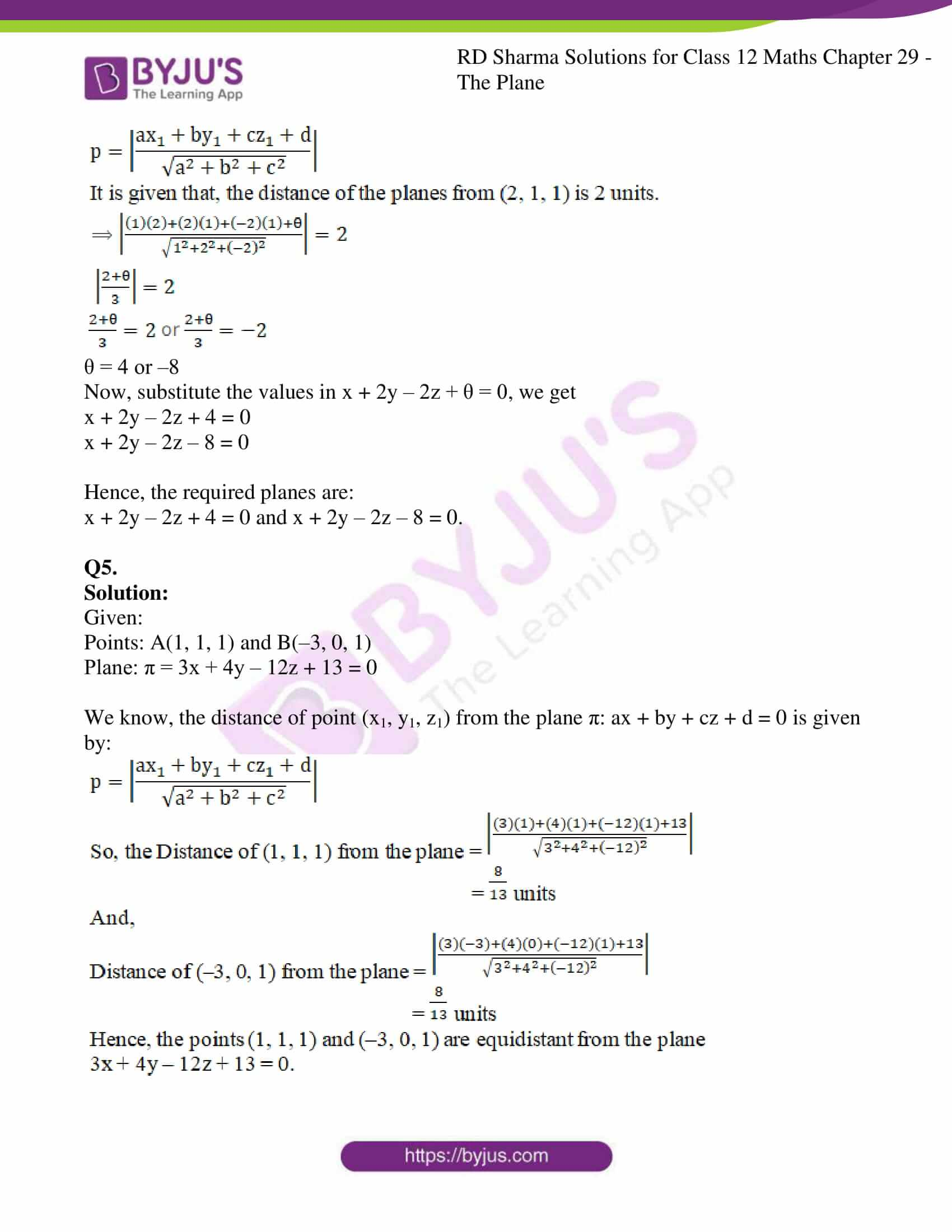### Access RD Sharma Solutions For Class 12 Maths Chapter 29 Exercise 9

EXERCISE 29.9

Q1.

Solution: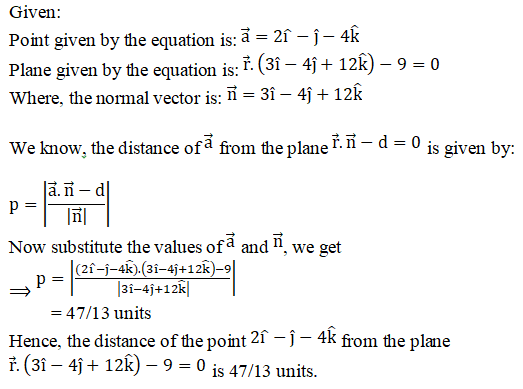Q2.

Solution: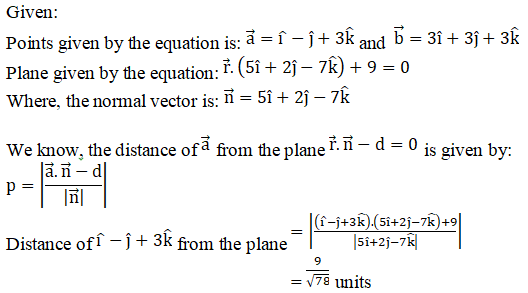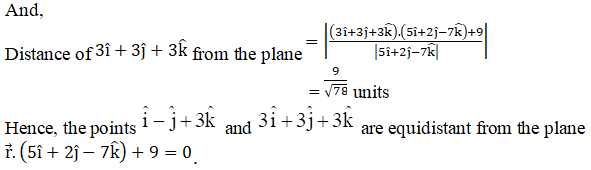Q3.

Solution:

Given:

Point: A(2, 3, –5)

Plane: π = x + 2y – 2z – 9 = 0

We know, the distance of point (x1, y1, z1) from the plane π: ax + by + cz + d = 0 is given by: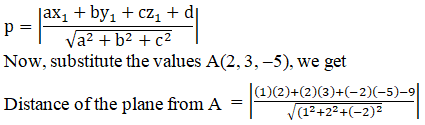= 3 units

Hence, the distance of the point (2, 3, –5) from the plane is 3 units.

Q4.

Solution:

Given:

The equation of plane is:

x + 2y – 2z + 8 = 0

Since the planes are parallel to x + 2y – 2z + 8 = 0, they must be of the form:

x + 2y – 2z + θ = 0

We know, the distance of point (x1, y1, z1) from the plane π: ax + by + cz + d = 0 is given by: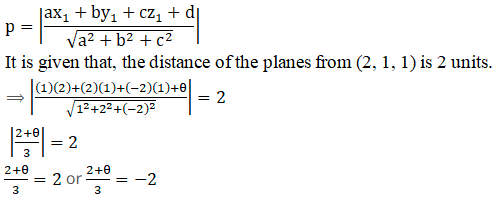θ = 4 or –8

Now, substitute the values in x + 2y – 2z + θ = 0, we get

x + 2y – 2z + 4 = 0

x + 2y – 2z – 8 = 0

Hence, the required planes are:

x + 2y – 2z + 4 = 0 and x + 2y – 2z – 8 = 0.

Q5.

Solution:

Given:

Points: A(1, 1, 1) and B(–3, 0, 1)

Plane: π = 3x + 4y – 12z + 13 = 0

We know, the distance of point (x1, y1, z1) from the plane π: ax + by + cz + d = 0 is given by: